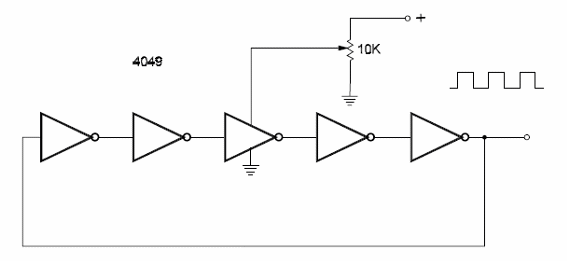DatasheetsN° of component

One-Component Oscillator (CB112)

Figure bellow shows a ring oscillator using five inverters of a 4049. [The circuit operates only with an odd number of gates] The frequency depends on the time delay of the signal when passing through each gate. Since this time depends on the power-supply voltage, a wide range of frequencies can be adjusted by the voltage divider in the power-supply input. With a power supply of 6 V, the circuit will generate signals typically in the range between 1 MHz and 4 MHz.One-Component Oscillator

Datasheet of 4049